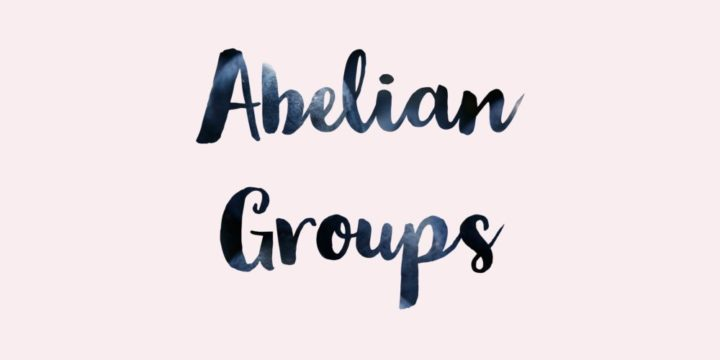# A Group is Abelian if and only if Squaring is a Group Homomorphism## Problem 325

Let $G$ be a group and define a map $f:G\to G$ by $f(a)=a^2$ for each $a\in G$.
Then prove that $G$ is an abelian group if and only if the map $f$ is a group homomorphism.Add to solve later

## Proof.

### $(\implies)$ If $G$ is an abelian group, then $f$ is a homomorphism.

Suppose that $G$ is an abelian group. We prove that $f:G \to G, a \mapsto a^2$ is a group homomorphism.
Let $a, b$ be arbitrary elements in $G$. Then we have
\begin{align*}
f(ab)&=(ab)^2 && (\text{ by definition of } f)\\
&=(ab)(ab)\\
&=a^2 b^2 && (\text{ since $G$ is abelian})\\
&=f(a)f(b) && (\text{ by definition of } f).
\end{align*}
Therefore, we obtain $f(ab)=f(a)f(b)$ for any $a, b\in G$.
Hence $f$ is a group homomorphism from $G$ to $G$.

### $(\impliedby)$ If $f$ is a homomorphism, then $G$ is an abelian group.

Suppose that $f:G\to G$ is a group homomorphism. We prove that $G$ is an abelian group.
Let $a, b\in G$. We want to prove that $ab=ba$.

Since $f$ is a group homomorphism, we have
$f(ab)=f(a)f(b).$ As a result we have
$(ab)^2=a^2b^2,$ or equivalently
$abab=aabb.$ Multiplying this by $a^{-1}$ on the left and by $b^{-1}$ on the right, we obtain
$ab=ba.$ Since $a$ and $b$ are arbitrary, this implies that $G$ is an abelian group.

## Related Question.

Another problem about the relation between an abelian group and a group homomorphism is:
A group homomorphism and an abelian group.Add to solve later

### 1 Response

1. 07/15/2017

[…] Another problem about the relation between an abelian group and a group homomorphism is: A group is abelian if and only if squaring is a group homomorphism […]

This site uses Akismet to reduce spam. Learn how your comment data is processed.

###### More in Group Theory##### The Additive Group $\R$ is Isomorphic to the Multiplicative Group $\R^{+}$ by Exponent Function

Let $\R=(\R, +)$ be the additive group of real numbers and let $\R^{\times}=(\R\setminus\{0\}, \cdot)$ be the multiplicative group of real...

Close